## Forced Duffing Oscillator

exb3
Variable and parameter:

u: state variable of an oscillator: frequency of the driving force (bifurcation parameter)

differential equation: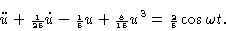We basically look for harmonic solutions, which are periodic with period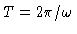.

Figure 1
Bifurcation diagram u(0) versus. Solid curve: branch of symmetric orbits.

Figure 2
Bifurcation diagramversus.

Figure 3
Detail of bifurcation diagram, u(0) versus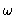.

Figure 4phase-plane for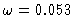, symmetric oscillation.

Figure 5
u(t) for, t is a normalized time, t=1 corresponds to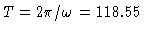.

Figure 6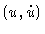plane for, asymmetric oscillation.

Figure 7
Bifurcation diagram of a closed branch of asymmetric solutions, u(0) versus.

Figure 8
Same as in Figure 7, but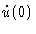versus.

Figure 9
Duffing equation,plane, Poincaré set of the stroboscopic map,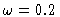, fractal dimension D=2.381.

This Example
Figure 1
Figure 2
Figure 3
Figure 4
Figure 5
Figure 6
Figure 7
Figure 8
Figure 9